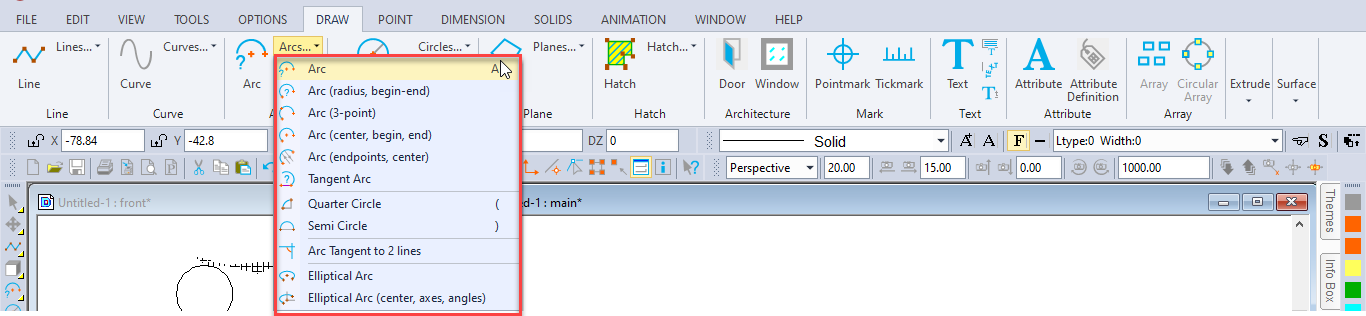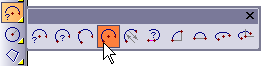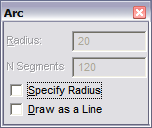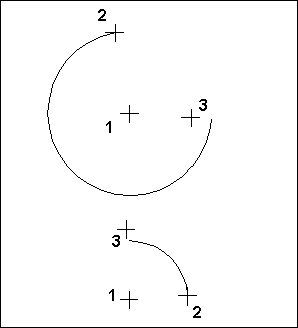# Arc(Center, Begin, End)Toolbox Icon:Draws an arc using the center, beginning, and end points of the arc.

Point 1: Center of arc

Point 2: Beginning of arc

Point 3: End of arc

There are two choices for the format of the arc.If you select Draw as a Line, the arc will be drawn as line segments that follow the shape of an arc. Otherwise the arc will be stored as an actual arc. If you Specify Radius, the radius is independent of the distance between the first and second points.

The arc format stores a center point, starting point, endpoint, and orientation point in the drawing. The line format stores a series of points for the line approximating the arc.

The first point you set becomes the center of the arc. The second point sets both the radius and the start angle of the arc. The third point sets the ending angle of the arc, and will not necessarily lie on the endpoint of the actual arc that is drawn.NOTE: Arcs drawn with this command are created in such a way that the arc can only span a positive angle from its starting point, so the arc will be drawn coun- terclockwise from point 2 to the span angle on the arc determined by point 3.

N Segments: When saved as a line, this is the number of segments, into which the object will be divided.